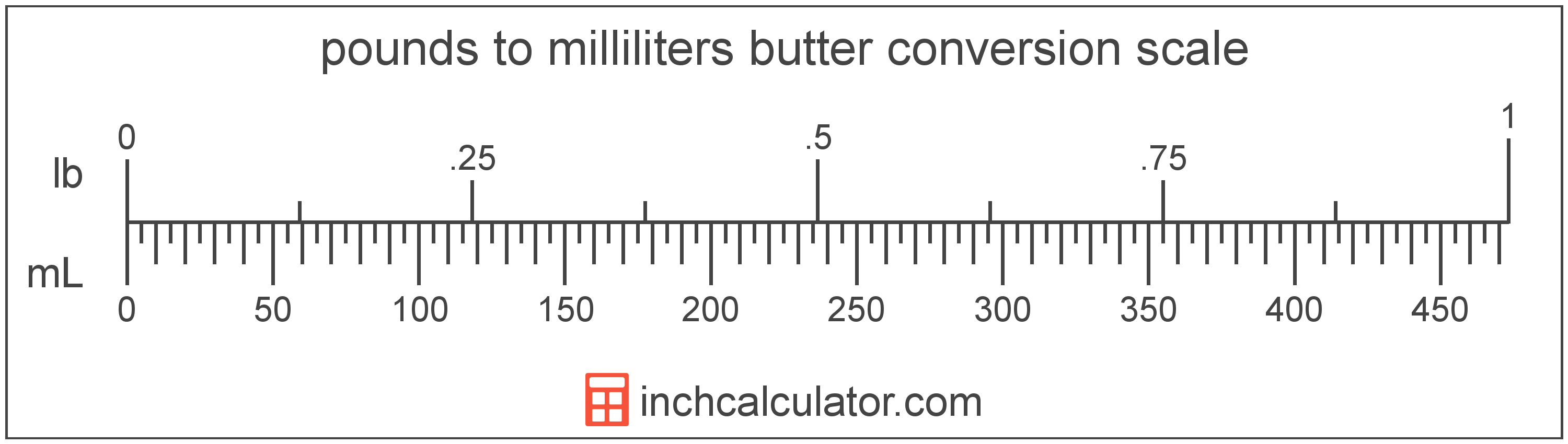# Convert Pounds of Butter to Milliliters

Enter the butter in pounds below to get the value converted to milliliters.

Results in Milliliters:1 lb = 473.17648 mL

Do you want to convert milliliters to pounds?

## How to Convert Pounds to Milliliters

To convert a measurement in pounds to a measurement in milliliters, multiply the butter by the following conversion ratio: 473.17648 milliliters/pound.

Since one pound of butter is equal to 473.17648 milliliters, you can use this simple formula to convert:

milliliters = pounds × 473.17648

The butter in milliliters is equal to the butter in pounds multiplied by 473.17648.

For example, here's how to convert 5 pounds to milliliters using the formula above.
milliliters = (5 lb × 473.17648) = 2,365.8824 mL### How Many Milliliters Are in a Pound of Butter?

There are 473.17648 milliliters in a pound of butter, which is why we use this value in the formula above.

1 lb = 473.17648 mL

## What is a Pound?

Butter in the US is most commonly sold by the pound, which contains 4 sticks, or 16 ounces.

The pound is a US customary and imperial unit of weight. Pounds can be abbreviated as lb; for example, 1 pound can be written as 1 lb.

## What is a Milliliter?

Butter is sometimes measured by volume in milliliters. One stick of butter contains about 118.3 milliliters.

The milliliter is an SI unit of volume in the metric system. A milliliter is sometimes also referred to as a millilitre. Milliliters can be abbreviated as mL; for example, 1 milliliter can be written as 1 mL.

## Pound to Milliliter Conversion Table

Table showing various pound measurements converted to milliliters.
Pounds Milliliters
1 lb 473.18 mL
2 lb 946.35 mL
3 lb 1,420 mL
4 lb 1,893 mL
5 lb 2,366 mL
6 lb 2,839 mL
7 lb 3,312 mL
8 lb 3,785 mL
9 lb 4,259 mL
10 lb 4,732 mL
11 lb 5,205 mL
12 lb 5,678 mL
13 lb 6,151 mL
14 lb 6,624 mL
15 lb 7,098 mL
16 lb 7,571 mL
17 lb 8,044 mL
18 lb 8,517 mL
19 lb 8,990 mL
20 lb 9,464 mL
21 lb 9,937 mL
22 lb 10,410 mL
23 lb 10,883 mL
24 lb 11,356 mL
25 lb 11,829 mL
26 lb 12,303 mL
27 lb 12,776 mL
28 lb 13,249 mL
29 lb 13,722 mL
30 lb 14,195 mL
31 lb 14,668 mL
32 lb 15,142 mL
33 lb 15,615 mL
34 lb 16,088 mL
35 lb 16,561 mL
36 lb 17,034 mL
37 lb 17,508 mL
38 lb 17,981 mL
39 lb 18,454 mL
40 lb 18,927 mL